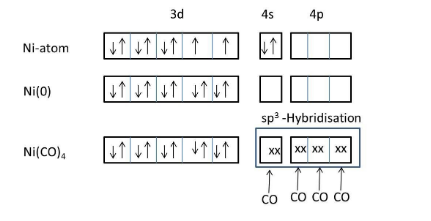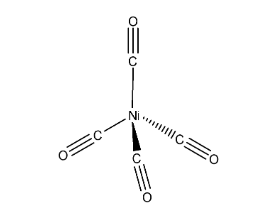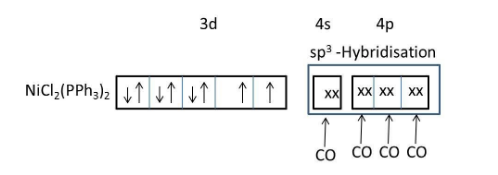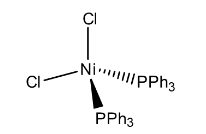QuestionAnswers

# The geometry of $Ni{{(CO)}_{4}}$and $Ni{{(PP{{h}_{3}})}_{2}}C{{l}_{2}}$are :(A) Both square planar(B) Tetrahedral and square planar, respectively(C) Both tetrahedral(D) Square planar and tetrahedral, respectively

Hint: $Ni{{(CO)}_{4}}$ and $Ni{{(PP{{h}_{3}})}_{2}}C{{l}_{2}}$ are examples of organometallic complexes. Nickel belongs to d-block elements. Nickel can form metallic complexes easily with ligands. Generally strong field ligands form tetrahedral complexes with nickel and weak field complexes form square planar complexes with nickel.

Complete step by step by answer:
The IUPAC name of $Ni{{(CO)}_{4}}$ is tetracarbonyl nickel (0).We know that carbonyl is a strong ligand, that’s why the two electrons in 4s orbital are shifted to 3d orbital of nickel.
Meanwhile no inner empty 3d orbital is available to form a bond with carbonyl ligand, 4s and 4p orbitals will take electrons from four carbonyl ligands and form four covalent bonds through the carbon end to the nickel atom.
The hybridization of nickel atoms in $Ni{{(CO)}_{4}}$ is$s{{p}^{3}}$.
The geometry of Nickel tetracarbonyl is tetrahedral.In the above structure it represents that the two carbonyls are in one plane (imagine x-axis), other two carbonyls are in different planes (y-axis and z-axis) to reduce the repulsions and then the structure will be tetrahedral.
IUPAC name of $Ni{{(PP{{h}_{3}})}_{2}}C{{l}_{2}}$is Dichlorobis(triphenylphosphine)nickel(II).$Ni{{(PP{{h}_{3}})}_{2}}C{{l}_{2}}$consists of both weak field ligand ($Cl$) and strong field ligand ($PP{{h}_{3}}$).
Presence of both weak field and strong field ligands $Ni{{(PP{{h}_{3}})}_{2}}C{{l}_{2}}$should exist in two geometries (tetrahedral and square planar).
But, due to the presence of larger ligands favoring the less crowded tetrahedral geometry.In the above structure it represents that the two chlorine atoms are in one plan (imagine x-axis), other two triphenylphosphine ligands are in different planes (y-axis and z-axis) to reduce the repulsions and then the structure will be tetrahedral.
Hence, the geometry of the Dichlorobis(triphenylphosphine)nickel(II) is tetrahedral.

So, the correct option is C.

Note: Don’t be confused with tetrahedral and square planar geometries. If the ligand forms bonds with 3d orbital of nickel then there is a chance of formation of square planar geometry.